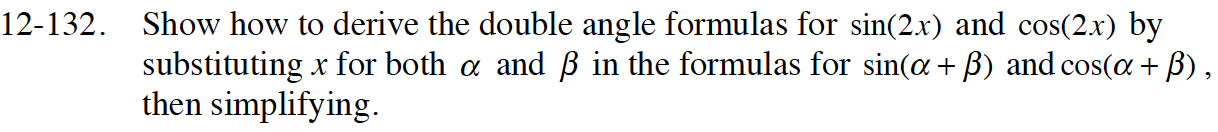Home > CCA2 > Chapter 12 > Lesson 12.2.3 > Problem12-132

12-132.

Show how to derive the double angle formulas for sin(2x) and cos(2x) by substituting x for both α and β in the formulas for sin(α + β) and cos(α + β), then simplifying. Homework Help ✎sin(x + x)

What is this equivalent to? How can you change it?

$\sin (x+x)\ =\ \sin (x)\cos (x)+\sin (x)\cos (x)\ =\ 2\sin (x)\cos (x)$

Now use the same method to find the the double angle formula for cos(2x).